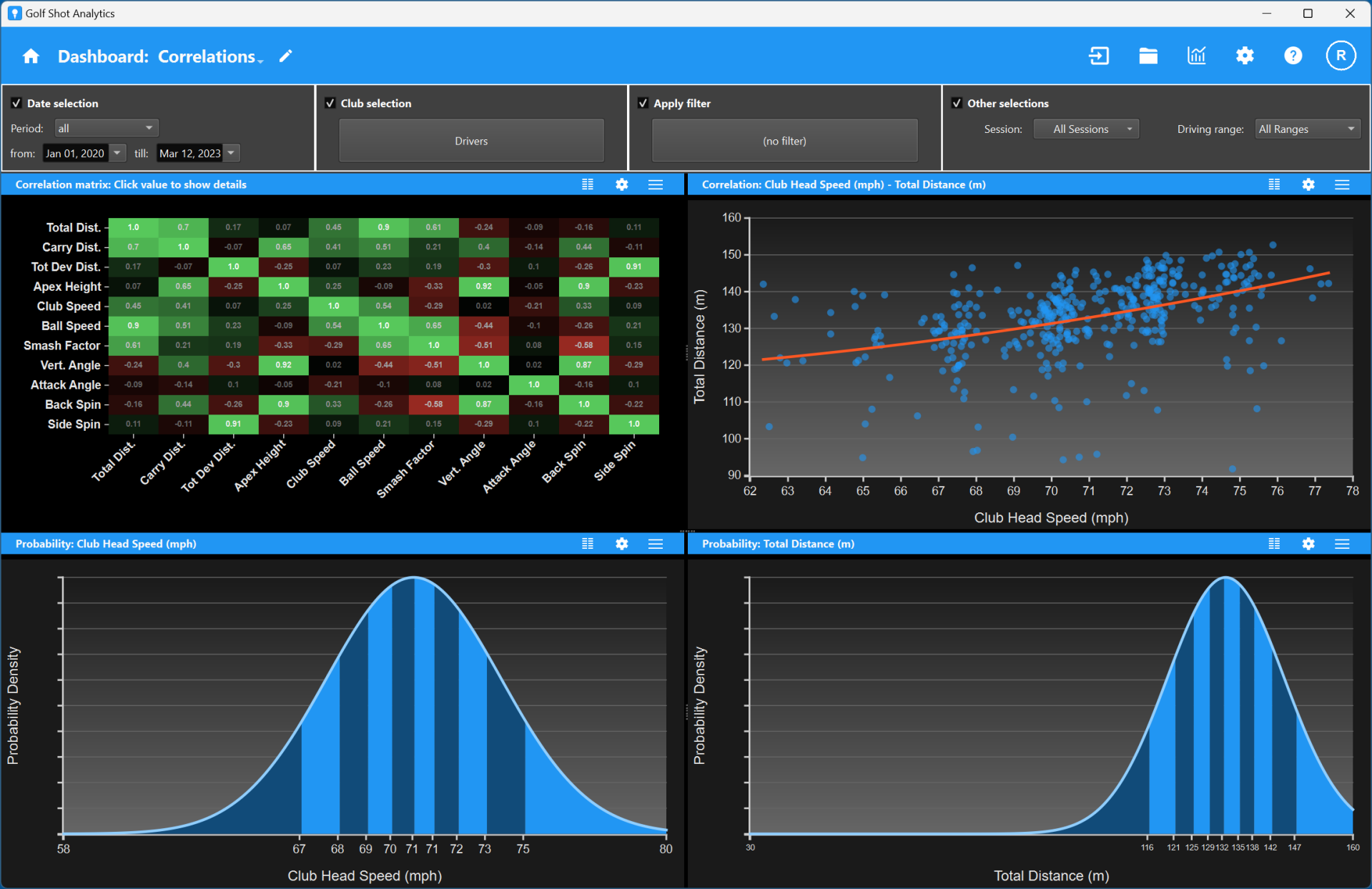## Correlations dashboardAn important piece of information is how certain metrics depend on each other. For example, what is the relation between the club head speed and the total distance? You expect the total distance to increase when you increase the club head speed, but at some point the ball contact might get worse and the total distance could decrease. The correlations dashboard is meant for this. It consist of four components:

• At the top-left there is a correlation matrix component. This shows you the correlations between pairs of metrics. A large value (close to 1) corresponds to a strong correlation, a low value (close to -1) to a strong negative correlations. Values close to 0 point to hardly any correlation. If you click on a correlation box in the matrix the other charts are adapted to those metrics.
• At the top-right there is a correlation chart component. It shows the correlation between two metrics. Each dot is a shot with that pair of values. The red curve shows the regression which is an indication of how the metrics depend on each other. The top (or bottom) of the red curve typically corresponds to the optimal combination.
• The bottom two distribution chart components show the probability distribution for the two metrics. This indicates the chance that your shots have a certain metric value. So while the correlation chart indicates the optimal combination, the distribution charts give information on how likely you are to get those values.

Note that you can always change the correlations dashboard if you prefer other component. For this, use the edit icon in the toolbar. You can also change the settings of each component to change the information shown and the look.